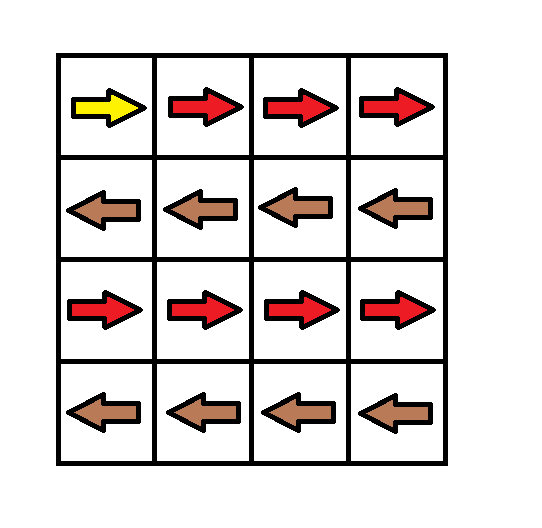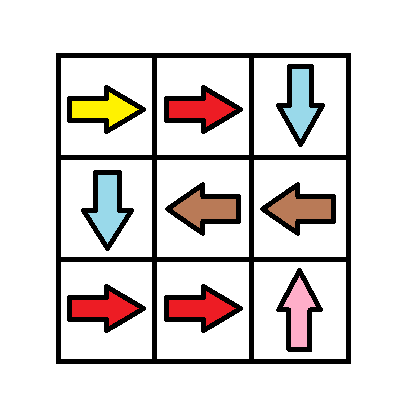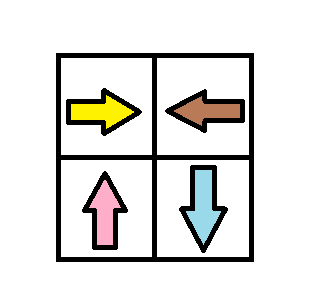Formatted question description: https://leetcode.ca/all/1368.html

# 1368. Minimum Cost to Make at Least One Valid Path in a Grid (Hard)

Given a m x n grid. Each cell of the grid has a sign pointing to the next cell you should visit if you are currently in this cell. The sign of grid[i][j] can be:

• 1 which means go to the cell to the right. (i.e go from grid[i][j] to grid[i][j + 1])
• 2 which means go to the cell to the left. (i.e go from grid[i][j] to grid[i][j - 1])
• 3 which means go to the lower cell. (i.e go from grid[i][j] to grid[i + 1][j])
• 4 which means go to the upper cell. (i.e go from grid[i][j] to grid[i - 1][j])

Notice that there could be some invalid signs on the cells of the grid which points outside the grid.

You will initially start at the upper left cell (0,0). A valid path in the grid is a path which starts from the upper left cell (0,0) and ends at the bottom-right cell (m - 1, n - 1) following the signs on the grid. The valid path doesn't have to be the shortest.

You can modify the sign on a cell with cost = 1. You can modify the sign on a cell one time only.

Return the minimum cost to make the grid have at least one valid path.

Example 1:Input: grid = [[1,1,1,1],[2,2,2,2],[1,1,1,1],[2,2,2,2]]
Output: 3
Explanation: You will start at point (0, 0).
The path to (3, 3) is as follows. (0, 0) --> (0, 1) --> (0, 2) --> (0, 3) change the arrow to down with cost = 1 --> (1, 3) --> (1, 2) --> (1, 1) --> (1, 0) change the arrow to down with cost = 1 --> (2, 0) --> (2, 1) --> (2, 2) --> (2, 3) change the arrow to down with cost = 1 --> (3, 3)
The total cost = 3.


Example 2:Input: grid = [[1,1,3],[3,2,2],[1,1,4]]
Output: 0
Explanation: You can follow the path from (0, 0) to (2, 2).


Example 3:Input: grid = [[1,2],[4,3]]
Output: 1


Example 4:

Input: grid = [[2,2,2],[2,2,2]]
Output: 3


Example 5:

Input: grid = []
Output: 0


Constraints:

• m == grid.length
• n == grid[i].length
• 1 <= m, n <= 100

Related Topics: Breadth-first Search

## Solution 1. BFS + DP

// OJ: https://leetcode.com/problems/minimum-cost-to-make-at-least-one-valid-path-in-a-grid/

// Time: O(MN)
// Space: O(MN)
class Solution {
public:
int minCost(vector<vector<int>>& G) {
int M = G.size(), N = G.size(), dp = {}, dirs = { {0,1},{0,-1},{1,0},{-1,0} };
memset(dp, 0x3f, sizeof(dp));
dp = 0;
queue<pair<int, int>> q;
q.emplace(0, 0);
while (q.size()) {
auto [x, y] = q.front();
q.pop();
for (int i = 0; i < 4; ++i) {
auto &[dx, dy] = dirs[i];
int a = x + dx, b = y + dy;
if (a < 0 || b < 0 || a >= M || b >= N) continue;
if (dp[x][y] + (G[x][y] - 1 != i) < dp[a][b]) {
dp[a][b] = dp[x][y] + (G[x][y] - 1 != i);
q.emplace(a, b);
}
}
}
return dp[M - 1][N - 1];
}
};


Java

class Solution {
int[][] directions = { {0, 1}, {0, -1}, {1, 0}, {-1, 0} };

public int minCost(int[][] grid) {
int rows = grid.length, columns = grid.length;
if (rows == 1 && columns == 1)
return 0;
int cost = 0;
boolean[][] visited = new boolean[rows][columns];
visited = true;
Queue<int[]> queue = new LinkedList<int[]>();
queue.offer(new int[]{0, 0});
List<int[]> levelList = new ArrayList<int[]>();
while (!queue.isEmpty()) {
int[] cell = queue.poll();
int row = cell, column = cell;
int directionIndex = grid[row][column] - 1;
if (directionIndex >= 0) {
int[] direction = directions[directionIndex];
int newRow = row + direction, newColumn = column + direction;
while (newRow >= 0 && newRow < rows && newColumn >= 0 && newColumn < columns && !visited[newRow][newColumn]) {
visited[newRow][newColumn] = true;
int nextDirectionIndex = grid[newRow][newColumn] - 1;
if (nextDirectionIndex < 0)
break;
newRow += directions[nextDirectionIndex];
newColumn += directions[nextDirectionIndex];
}
} else {
for (int i = 0; i < 4; i++) {
int newRow = row + directions[i], newColumn = column + directions[i];
while (newRow >= 0 && newRow < rows && newColumn >= 0 && newColumn < columns && !visited[newRow][newColumn]) {
visited[newRow][newColumn] = true;
int nextDirectionIndex = grid[newRow][newColumn] - 1;
if (nextDirectionIndex < 0)
break;
newRow += directions[nextDirectionIndex];
newColumn += directions[nextDirectionIndex];
}
}
}
if (queue.isEmpty() && !levelList.isEmpty()) {
for (int[] levelCell : levelList) {
int curRow = levelCell, curColumn = levelCell;
if (curRow == rows - 1 && curColumn == columns - 1)
return cost;
queue.offer(levelCell);
grid[curRow][curColumn] = -1;
}
cost++;
levelList.clear();
}
}
return cost;
}
}